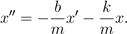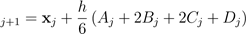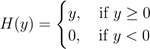# We Helped With This Differential Equations Assignment: Have A Similar One?

SOLVEDCategory Math Differential Equations College Solved Differential Equations Help

## Short Assignment Requirements

details are provided in the documents.One side not is it says in 1a that there a code for Euler method provided but is not the case and one needs to be made from the beginning

## Assignment Description

Lab Project Week 7: Second order equations, systems of Equations and Runge-

Kutta methods

In the last project, you coded up Euler’s method for scalar first order equations:

(1)                                                                                                       x0 = f(t,x),            x(t0) = x0.

In class you are currently considering second order scalar equations which have the form

(2)                                                                                                       x00 = f(t,x,x0),      x(t0) = x0 and  x0(t0) = v0.

For instance, the equation for a damped harmonic oscillator looks likeSo how do we fix up EM to handle the “second derivative” which appears in the above? The answer is to convert the second order scalar equation into a pair of first order equations.

Conversion from second order scalar equation to a first order system: Let v(t) = x0(t). Then observe that the differential equation in (2) is the same as v0 = f(t,x,v). So all together we have

x0 = v

(3)                                                                                                       v0 = f(t,x,v)

x(t0) = x0 and        v(t0) = v0.

In the above only first derivatives appear. If we put x = (x,v), f(t,x) = (v,f(t,x,v)) and x0 = (x0,v0) then we can compress the above system to

(4)                                                                                                       x0 = f(t,x),           x(t0) = x0.

This looks a lot like (1) except now x and f are vectors in R2 instead of scalars. So now you apply EM, but adjusted to handle vectors instead of scalars.

EM for the system (4): Pick h R kind of small. Note that in (4) you are given t0 and x0. For integers j ≥ 0 put

(5)                                                                                                       tj+1 = tj + h           and      xj+1 = xj + hf(tj,xj).

Then, if h is small enough, one expects

x(tj) ∼ xj.

That is to say, the true solution of (4) is approximated at t = tj by xj.

Problem 1: Adjust your EM code along the lines described above to simulate solutions of the equation

(6)                                                                                                       x00 = −x,  x(0) = 1           and      x0(0) = 0

for t ∈ [0,20]. Note that the true solution of this equation is x(t) = cos(t). Run your code for h = 1,1/4,1/16,1/64 and compare the output with the true solution.

What you will find is that, even for small values of h the numerical method does not work particularly well. The approximation will always grow in amplitude while the true solution does not. The reason for this is that Euler’s Method is not really very accurate. A better method, and one that is widely used in industry, is called “Fourth-Order Runge-Kutta” or “RK4”.

1

2

RK4 for the system (4): Pick h R sort of small. Note that in (4) you are given t0 and x0. For integers j ≥ 0 put

(7)                          tj+1 = tj + h      and    xwhere

(8)Then, as in EM, we have x(tj) ∼ xj.

The error |x(tj) − xj| will be smaller, for the same h, if you use RK4 vs EM, and that is the point of the next problem.

Problem 2: Implement RK4 for (6) for t ∈ [0,20]. Run your code for h = 1,1/4,1/16,1/64 and compare the output with the true solution and the output from EM (with the same step size). You will see that RK4 is remarkably better even at very large step sizes.

At this point you now have a RK4 solver and the ability to apply it to second order scalar equations. So let’s do it.

Problem 3: Implement RK4 for the “ideal pendulum equation”

x00 = −sin(x),         x(0) = x0 and       x0(0) = v0.

This is the equation of motion for a rigid pendulum of length 1, with mass equal to 1, in a place where the acceleration due to gravity is exactly 1 and there is no friction. Here x is the angle the pendulum makes with respect to vertical. Your TA will draw a picture for you.

Use your solver to simulate the above with the following initial conditions. For each, describe in words (to each other and to your TA) what is going on with pendulum.

x0 = 0, v0 = 0. • x0 = 0, v0 = 1.

x0 = 0, v0 = 2.

x0 = 0, v0 = 2.1.

x0 = π, v0 = 0.

x0 = π, v0 = 0.1.

Use a step size of h = 1/128 and simulate each on the interval t = [0,50].

 It might seem that RK4 is pretty strange; why it works is beyond the scope of this class but probably not beyond your understanding. It has something to do with Simpson’s rule for approximating integrals: you should read about it if you are curious. The Wikipedia page on Runge-Kutta is a pretty good reference.

## Assignment Description

Lab Project 2 (Week 8): Resonance and the Tacoma Narrows Bridge

You have been extensively studying driven simple harmonic oscillators, which are modeled by the equation

(1)                                                       mx00 + cx0 + kx = F(t).

Here, x is the dispacement of the oscillator from equilbrium, m is the mass, c is the damping constant, k is the spring constant and F(t) is the driving force. This project is about resonance: at resonant frequencies, small periodic driving forces have the ability to produce large amplitude oscillations, due to the storage of vibrational energy.

No engineering student gets through their life without being told that resonance is why the Tacoma Narrows Bridge (TNB) fell over. If you don’t know what the TNB is, then get on your phone right now, go to YouTube and type “Tacoma Narrows Bridge” into the search bar and watch some videos. In this project, you are going to do some numerical experiments for a (slightly!) more sophisticated model of the TNB.

Here’s the standard story about the TNB: wind blew across the bridge and the frequency of the oscillations of the wind was close to the natural frequency of the bridge. Hence there was resonance, which caused the oscillations of the bridge to get huge which tore it apart. It’s a good story, and not totally wrong. But, obviously, a bridge is not a mass on a spring. So here we’ll study a nonlinear modification of (1), developed by Lazer & McKenna in 1990,which some people feel gives an at least slightly more realistic model for TNB.

Here is the idea: the cables on a suspension bridge only exert a force when they are stretched, not when they are slack. The spring/mass system modeled by (1) has a spring which pushes back when it is compressed and pulls when stretched. To “fix up” (1) to be more like a suspension bridge, Lazer & McKenna proposed using the following equation:

(2)                                            y00 + by0 + k1y + k2H(y) = 10 + F(t).

In the above y is the horizontal displacement of the bridge, measured “downward.” That is to say, if y > 0 then the bridge has moved closer to the ground. Note that we are treating the whole bridge as one piece, a pretty big assumption! The constant b is the damping constant. The number “10” is meant to be the acceleration due to gravity. The constants k1 and k2 are spring constants. Again, F(t) is a forcing function. Lastly,.

Note that H is only “on” if y ≥ 0 (which is “down”). If y < 0, which is to say if the bridge is elevated, then it exerts no force. Which is to say, it models slightly more realistically how the cables would exert force.

2

It turns out this model has an interesting feature which is that there are large amplitude steady state solutions even when the driving force is not at the resonant frequency. And you will investigate this now.

Problem 1: Modify the RK4 code you wrote in last project to simulate second order systems so that it works for (2). Keep it flexible enough so that you can easily change the constants k1, k2 and b, the initial conditions and the forcing function F(t). You might find the “heaviside” function to be useful in getting MATLAB to implement the function H(y). (Use a step size h = 1/16 throughout this project.)

Problem 2: Put k2 = 0, k1 = 17 and b = 0.01. Note that here the “nonlinear part” is zeroed out, so this is really just a driven simple harmonic oscillator. Suppose that F(t) = 0.1cos(ωt). Use the approach of Section 4.2 in the textbook to find the general solution, and describe its steady- state behavior (see the definition in Section 4.4).

The presence of the “10” on the right hand side of (2) will slightly complicate things, but a simple trick reduces the problem to exactly the form in the book. The trick is this: let y = C + z where z is a new function and C is cleverly chosen constant. Your TA should be able to help you with figure out this special constant! It has something to do with

“equilbrium.”

Problem 3: Put k2 = 0, k1 = 17 and b = 0.01. Put y(0) = 0 and y0(0) = 0. Let

F(t) = 0.1cos(ωt). For ω ∈ [3,5] (with increments of 0.1), run your solver from Problem 1 from t = 0 to t = 1500. This should be enough time for the solution to settle into its “steady-periodic state.” Compare your numerically computed solution with the formula for the steady state you got in Problem 2. They should be in pretty close agreement when t is large (but necessarily near t = 0). At what value of ω is the amplitude of the steady state biggest? This is, of course, the resonant frequency!

Problem 4: Put k2 = 0, k1 = 17 and b = 0.01. Let F(t) = 0.1cos(4t). You can determine from the formulas in 2.6 choices for y(0) and y0(0) so that the “transient solution” is exactly zero. Which is to say, you won’t need to “wait” to see that steady state part. Use these special initial conditions in the RK4 solver. Run your solver and see if your RK4 method with these special initial conditions and see if it agrees with the analytic solution (it should agree almost exactly instantly).

Problem 5: Take your special initial conditions from the last problem and use them as the initial conditions for the system with k2 = 5, k1 = 12, b = 0.01 and F(t) = 0.1cos(4t). If you have done things correctly, NOTHING should change from the previous problem. Why? This solution is called a “small amplitude periodic solution.”

Problem 6: With the constants and F(t) as in Problem 5, vary y(0) between −6 and 0 (with increments of 0.1) and y0(0) between 0 and 1 (again with increments of 0.1). Let your solver run from t = 0 to t = 1500 and record the amplitude of the solution at that time. Some of the initial conditions will have reach a steady state which is exactly the same small amplitude solution you saw in Problem 4. Other ones will be much larger in amplitude: these are “large amplitude steady states” and a nonlinear phenomenon, not a linear one. (That is to say, if you put k2 = 0 there is only the small amplitude solution.) Make a plot of the resulting steady state amplitude vs y(0) and y0(0). It is conjectured that that these sort of “large amplitude nonlinear periodic” solutions are the real culprit behind the TNB disaster! The point is: if the initial condition is set is just so, the solution is attracted to a

3

very large frequency oscillation even when the driving frequency is not right on the resonant frequency. NONLINEARITY = DOOM.

 This project is largely based on—and in some parts wholly borrowed from—Chapter 4.5 of Differential Equations by Blanchard, Devaney and Hall.

 Lazer & McKenna, “Large-amplitude periodic oscillations in suspension bridges: some new connections with nonlinear analysis” SIAM Review, 1990 pp 537-587

 Clearly civil engineers do way more sophisticated stuff than this when they study bridges! So if you really want a deep understand of TNB, take a class in that department!

 Mathematicians are lazy and can’t be bothered getting accurate physical constants; of course engineers know that acceleration due to gravity is really 9.807. But 10 is close to 9.807 so we’ll stick with it.

Is it free to get my assignment evaluated?

Yes. No hidden fees. You pay for the solution only, and all the explanations about how to run it are included in the price. It takes up to 24 hours to get a quote from an expert. In some cases, we can help you faster if an expert is available, but you should always order in advance to avoid the risks. You can place a new order here.

How much does it cost?

The cost depends on many factors: how far away the deadline is, how hard/big the task is, if it is code only or a report, etc. We try to give rough estimates here, but it is just for orientation (in USD):

 Regular homework \$20 - \$150 Advanced homework \$100 - \$300 Group project or a report \$200 - \$500 Mid-term or final project \$200 - \$800 Live exam help \$100 - \$300 Full thesis \$1000 - \$3000

How do I pay?

Credit card or PayPal. You don't need to create/have a Payal account in order to pay by a credit card. Paypal offers you "buyer's protection" in case of any issues.

Why do I need to pay in advance?

We have no way to request money after we send you the solution. PayPal works as a middleman, which protects you in case of any disputes, so you should feel safe paying using PayPal.

Do you do essays?

No, unless it is a data analysis essay or report. This is because essays are very personal and it is easy to see when they are written by another person. This is not the case with math and programming.

Why there are no discounts?

It is because we don't want to lie - in such services no discount can be set in advance because we set the price knowing that there is a discount. For example, if we wanted to ask for \$100, we could tell that the price is \$200 and because you are special, we can do a 50% discount. It is the way all scam websites operate. We set honest prices instead, so there is no need for fake discounts.

Do you do live tutoring?

No, it is simply not how we operate. How often do you meet a great programmer who is also a great speaker? Rarely. It is why we encourage our experts to write down explanations instead of having a live call. It is often enough to get you started - analyzing and running the solutions is a big part of learning.

What happens if I am not satisfied with the solution?

Another expert will review the task, and if your claim is reasonable - we refund the payment and often block the freelancer from our platform. Because we are so harsh with our experts - the ones working with us are very trustworthy to deliver high-quality assignment solutions on time.

Customer Feedback

"Thanks for explanations after the assignment was already completed... Emily is such a nice tutor! "

Order #13073